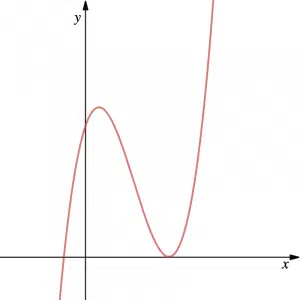# Algebra and Functions in A-Level Maths

## Algebra and Functions

Algebra and Functions are fundamental to most aspects of Pure Maths. For this reason, especially in the first year, this is one of the more important sections and we cover a relatively large number of topics.At GCSE, we learn about surds and indices and a large emphasis is put on introducing quadratics (see more about GCSE Maths). In particular, we learn to solve quadratics by factorising, using the quadratic formula or by completing the square. We also start to learn how to solve simultaneous equations and inequalities. At A-Level, we develop these areas first seen at GCSE and we learn how to use the discriminant of a quadratic. We also advance our knowledge of Algebra and Functions by looking at other polynomials such as cubics. Curve Sketching and Transformations of graphs (not necessarily just quadratics and cubics) are also an important part of Algebra & Functions. In the second year, we introduce a new function: the modulus function and we learn how to invert and compound functions.

The following lists of topics show the areas covered in the first year  (AS Maths) and in the second year (A2 Maths) of A-Level Maths or equivalent. Click on the links to look at our lessons in more detail.